Definitions of Square Dance Calls and Concepts

Index -->  Plus  |  A1  |  A2  |  C1  |  C2  |  C3A  |  C3B  |  C4  |  NOL  |
Definitions (Text Only) -->  Plus  |  A1  |  A2  |  C1  |  C2  |  C3A  |  C3B  |  C4  |  NOL  |
 Find call:

Reverse Checkpoint anything By anything -- [C3B]
(Vic Ceder 1985)

 \$B8@8l(B view (admin)

\$BE,@Z\$J(B formation \$B\$+\$i(B.

Center 4 \$B\$,;O\$a\$N(B anything \$B\$N%3!<%k\$r9T\$\$(B, \$B\$=\$N(B 4 \$B?M\$G(B Ends \$B\$H\$J\$C\$?(B \$B?M\$O(B, \$B8~\$-\$rJQ\$(\$:\$K(B (\$B\$9\$J\$o\$A(B Stable \$B\$G(B) Set \$B\$N(B Center \$B\$+\$iN%\$l\$^\$9(B. \$BB>\$N?M(B (\$B%*%j%8%J%k\$N30\$N?M(B) \$B\$O(B, Very Centers \$B\$N2s\$j\$G(B 2 \$B\$DL\\$N(B anything \$B\$N%3!<%k\$r9T\$\$(B, Center 4 \$B\$N(B Ends \$B\$H\$J\$C\$??M\$,6u\$1\$?>l=j\$X2DG=\$J8B\$j6a\$E\$-\$^\$9(B.

Reverse Checkpoint Recycle By Touch 1/4: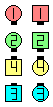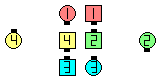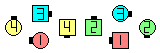Reverse CheckpointRecycle By Touch 1/4\$B\$NA0(B Centers Recycle and the resulting Ends slide away from the center\$B\$N8e(B original Outsides work aroundthe Very Centers to do a Touch 1/4\$B\$N8e(B (\$B=*\$o\$j(B)
Reverse Checkpoint 1/4 Thru By Follow Thru: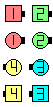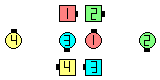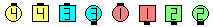Reverse Checkpoint1/4 Thru By Follow Thru\$B\$NA0(B Centers 1/4 Thru and the resulting Ends slide away from the center\$B\$N8e(B original Outsides work aroundthe Very Centers to do a Follow Thru\$B\$N8e(B (\$B=*\$o\$j(B)

\$B%@%s%9\$N%R%s%H(B: \$B%*%j%8%J%k\$N30\$N?M\$O(B, \$BIaDL(B, Centers \$B\$,;O\$a\$N(B anything \$B\$N%3!<%k\$r\$[\$\=*\$(\$k\$^\$GBT\$D\$Y\$-\$G\$9(B. \$B\$=\$&\$9\$l\$P(B, \$B30B&\$N?M\$K\$H\$C\$F(B, 2 \$B\$DL\\$N(B anything \$B\$N%3!<%k\$r\$9\$k>l=j\$,==J,\$K\$G\$-\$^\$9(B.

\$BCm

Checkpoint anything By anything [C2] (Lee Kopman 1982): 1 x 8, Point-to-Point Diamonds, \$B\$^\$?\$OB>\$N\$\$\$m\$\$\$m\$JE,@Z\$J(B formation \$B\$G!V(Beach side \$B\$K(B Centers \$B\$,\$\$\$k\$H\$3\$m!W\$+\$i(B. Each side \$B\$N(B Centers \$B\$,(B, \$B\$*8_\$\$\$K0l=o\$K(B (\$BH?BPB&\$N(B Centers \$B\$H(B) \$BF0\$-(B, concentric \$B\$K(B, Very Centers \$B\$N2s\$j\$G(B, \$B;O\$a\$N(B anything \$B\$N%3!<%k\$r9T\$\$\$^\$9(B (\$B\$b\$72DG=\$J\$i(B, \$B;O\$a\$ND9<4\$KD>8r\$9\$k>l=j\$X9T\$-\$^\$9(B). \$BBT\$C\$F\$\$\$?30B&\$N(B 2 \$B?M\$^\$?\$O(B 4 \$B?M\$O(B, Center \$B\$XF0\$-(B (\$B8~\$-\$rJQ\$(\$:\$K(B), \$B%*%j%8%J%k\$N(B Very Centers \$B\$H0l=o\$K(B, 2 \$B\$DL\\$N(B anything \$B\$N%3!<%k\$r9T\$\$\$^\$9(B. Checkpoint anything By anything [C2]Choreography for Reverse Checkpoint anything By anythingComments? Questions? Suggestions?

https://www.ceder.net/def/reversecheckpoint.php?level=master&language=japan
21-June-2021 21:48:43
Copyright © 2021 Vic Ceder.  All Rights Reserved.